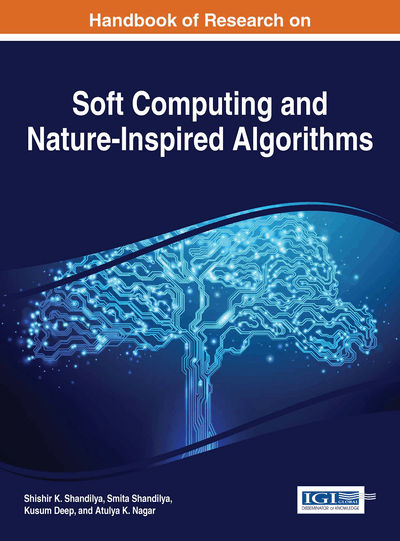# Augmented Lagrange Hopfield Network for Combined Economic and Emission Dispatch with Fuel Constraint

Vo Ngoc Dieu (Ho Chi Minh City University of Technology, Vietnam) and Tran The Tung (Ho Chi Minh City University of Technology, Vietnam)
DOI: 10.4018/978-1-5225-2128-0.ch007

## Abstract

This chapter proposes an augmented Lagrange Hopfield network (ALHN) for solving combined economic and emission dispatch (CEED) problem with fuel constraint. In the proposed ALHN method, the augmented Lagrange function is directly used as the energy function of continuous Hopfield neural network (HNN), thus this method can properly handle constraints by both augmented Lagrange function and sigmoid function of continuous neurons in the HNN. For dealing with the bi-objective economic dispatch problem, the slope of sigmoid function in HNN is adjusted to find the Pareto-optimal front and then the best compromise solution for the problem will be determined by fuzzy-based mechanism. The proposed method has been tested on many cases and the obtained results are compared to those from other methods available the literature. The test results have shown that the proposed method can find good solutions compared to the others for the tested cases. Therefore, the proposed ALHN could be a favourable implementation for solving the CEED problem with fuel constraint.
Chapter Preview
Top

## Nomenclature

• ai, bi, ci emission coefficients for thermal unit i

• di, ef, fi fuel cost coefficients for thermal unit i

• Bij, B0i, B00 transmission loss formula coefficients

• Fik fuel delivery for thermal unit i during subinterval k, in tons

• Fimin, Fimax lower and upper fuel delivery limits for thermal unit i, in tons

• M number of subintervals of scheduled period

• N total number of thermal units;

• PDk load demand of the system during subinterval k, in MW

• PLk transmission loss of the system during subinterval k, in MW

• Pik output power of thermal unit i during subinterval k, in MW

• Pimin, Pimax lower and upper generation limits of thermal unit i, in MW

• Qik fuel consumption function of thermal unit i in subinterval k, in tons/h

• tk duration of subinterval k, in hours

• Xi0 initial fuel storage for unit i, in tons

• Xik fuel storage for unit i during subinterval k, in tons

• Ximin, Ximax lower and upper fuel storage limits for thermal unit i, in tons

• Vp,ik output of continuous neuron representing for output power Pik

• Vf,ik output of continuous neuron representing for fuel delivery Fik

• Vx,ik output of continuous neuron representing for fuel storage Xik

• Vλ,k output of multiplier neuron associated with power balance constraint

• Vγ,k output of multiplier neuron associated with fuel delivery constraint

• Vη,ik output of multiplier neuron associated with fuel storage constraint

• Up,ik, Uf,ik, Ux,ik inputs of continuous neurons corresponding to the outputs Vp,ik, Vf,ik and Vx,ik, respectively

• Uλ,k, Uγ,k, Uη,ik inputs of multiplier neurons corresponding to the outputs Vλ,k, Vγ,k and Vη,ik, respectively

• ΔPk power balance constraint error in sub-interval k, in MW

• ΔFk fuel delivery constraint error in subinterval k, in tons

• ΔXik fuel storage constraint error for unit i during subinterval k, in tons

• ΔVp,ik, ΔVf,ik, ΔVx,ik iterative errors of continuous neurons

• σ slope of sigmoid function of continuous neurons

• αp, αf, αx updating step sizes for continuous neurons

• αλ, αγ, αη updating step sizes for multiplier neurons

• φ0i, φ1i, φ2i fuel consumption coefficients for thermal unit i

• λk, γk, ηik Lagrangian multipliers associated with power balance, fuel delivery and fuel storage constraints, respectively

• βλ,k, βγ,k, βη,sk penalty factors associated with power balance, fuel delivery and fuel storage constraints, respectively

## Complete Chapter List

Search this Book:
Reset